JMSLTM Numerical Library 7.2.0
com.imsl.stat

Class AutoCorrelation

• All Implemented Interfaces:
Serializable, Cloneable

public class AutoCorrelation
extends Object
implements Serializable, Cloneable
Computes the sample autocorrelation function of a stationary time series.

AutoCorrelation estimates the autocorrelation function of a stationary time series given a sample of n observations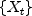for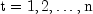.

Let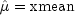be the estimate of the mean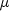of the time serieswhere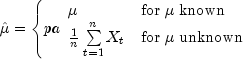The autocovariance function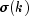is estimated by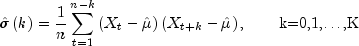where K = maximum_lag. Note that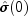is an estimate of the sample variance. The autocorrelation function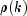is estimated by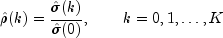Note that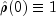by definition.

The standard errors of sample autocorrelations may be optionally computed according to the getStandardErrors method argument stderrMethod. One method (Bartlett 1946) is based on a general asymptotic expression for the variance of the sample autocorrelation coefficient of a stationary time series with independent, identically distributed normal errors. The theoretical formula is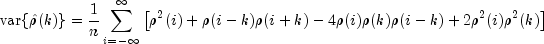where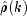assumesis unknown. For computational purposes, the autocorrelationsare replaced by their estimatesfor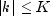, and the limits of summation are bounded because of the assumption that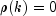for allsuch that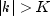.

A second method (Moran 1947) utilizes an exact formula for the variance of the sample autocorrelation coefficient of a random process with independent, identically distributed normal errors. The theoretical formula is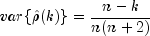whereis assumed to be equal to zero. Note that this formula does not depend on the autocorrelation function.

The method getPartialAutoCorrelations estimates the partial autocorrelations of the stationary time series given K = maximum_lag sample autocorrelationsfor k=0,1,...,K. Consider the AR(k) process defined by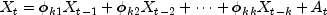where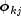denotes the j-th coefficient in the process. The set of estimates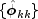for k = 1, ..., K is the sample partial autocorrelation function. The autoregressive parameters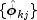for j = 1, ..., k are approximated by Yule-Walker estimates for successive AR(k) models where k = 1, ..., K. Based on the sample Yule-Walker equations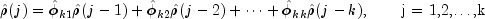a recursive relationship for k=1, ..., K was developed by Durbin (1960). The equations are given by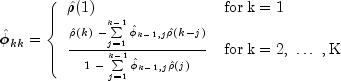and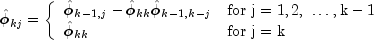This procedure is sensitive to rounding error and should not be used if the parameters are near the nonstationarity boundary. A possible alternative would be to estimate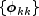for successive AR(k) models using least or maximum likelihood. Based on the hypothesis that the true process is AR(p), Box and Jenkins (1976, page 65) note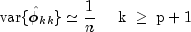See Box and Jenkins (1976, pages 82-84) for more information concerning the partial autocorrelation function.

Example, Serialized Form
• Field Summary

Fields
Modifier and Type Field and Description
static int BARTLETTS_FORMULA
Indicates standard error computation using Bartlett's formula.
static int MORANS_FORMULA
Indicates standard error computation using Moran's formula.
• Constructor Summary

Constructors
Constructor and Description
AutoCorrelation(double[] x, int maximum_lag)
Constructor to compute the sample autocorrelation function of a stationary time series.
• Field Detail

• BARTLETTS_FORMULA

public static final int BARTLETTS_FORMULA
Indicates standard error computation using Bartlett's formula.
Constant Field Values
• MORANS_FORMULA

public static final int MORANS_FORMULA
Indicates standard error computation using Moran's formula.
Constant Field Values
• Constructor Detail

• AutoCorrelation

public AutoCorrelation(double[] x,
int maximum_lag)
Constructor to compute the sample autocorrelation function of a stationary time series.
Parameters:
x - a one-dimensional double array containing the stationary time series
maximum_lag - an int containing the maximum lag of autocovariance, autocorrelations, and standard errors of autocorrelations to be computed. maximum_lag must be greater than or equal to 1 and less than the number of observations in x
• Method Detail

• getAutoCorrelations

public double[] getAutoCorrelations()
Returns the autocorrelations of the time series x.
Returns:
a double array of length maximum_lag +1 containing the autocorrelations of the time series x. The 0-th element of this array is 1. The k-th element of this array contains the autocorrelation of lag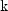where k = 1, ..., maximum_lag.
• getAutoCovariances

public double[] getAutoCovariances()
throws AutoCorrelation.NonPosVariancesException
Returns the variance and autocovariances of the time series x.
Returns:
a double array of length maximum_lag +1 containing the variances and autocovariances of the time series x. The 0-th element of the array contains the variance of the time series x. The k-th element contains the autocovariance of lag k where k = 1, ..., maximum_lag.
Throws:
AutoCorrelation.NonPosVariancesException - is thrown if the problem is ill-conditioned
• getMean

public double getMean()
Returns the mean of the time series x.
Returns:
a double containing the mean

Returns the number of java.lang.Thread instances used for parallel processing.
Returns:
an int containing the number of java.lang.Thread instances used for parallel processing.
• getPartialAutoCorrelations

public double[] getPartialAutoCorrelations()
Returns the sample partial autocorrelation function of the stationary time series x.
Returns:
a double array of length maximum_lag containing the partial autocorrelations of the time series x.
• getStandardErrors

public double[] getStandardErrors(int stderrMethod)
Returns the standard errors of the autocorrelations of the time series x. Method of computation for standard errors of the autocorrelation is chosen by the stderrMethod parameter. If stderrMethod is set to BARTLETTS_FORMULA, Bartlett's formula is used to compute the standard errors of autocorrelations. If stderrMethod is set to MORANS_FORMULA, Moran's formula is used to compute the standard errors of autocorrelations.
Parameters:
stderrMethod - an int specifying the method to compute the standard errors of autocorrelations of the time series x
Returns:
a double array of length maximum_lag containing the standard errors of the autocorrelations of the time series x
• getVariance

public double getVariance()
Returns the variance of the time series x.
Returns:
a double containing the variance of the time series x
• setMean

public void setMean(double mean)
Estimate mean of the time series x.
Parameters:
mean - a double containing the estimate mean of the time series x.